# Maclaurin Spheroid Sequence

## Detailed Force Balance Conditions

### Equilibrium Angular Velocity

 Figure 1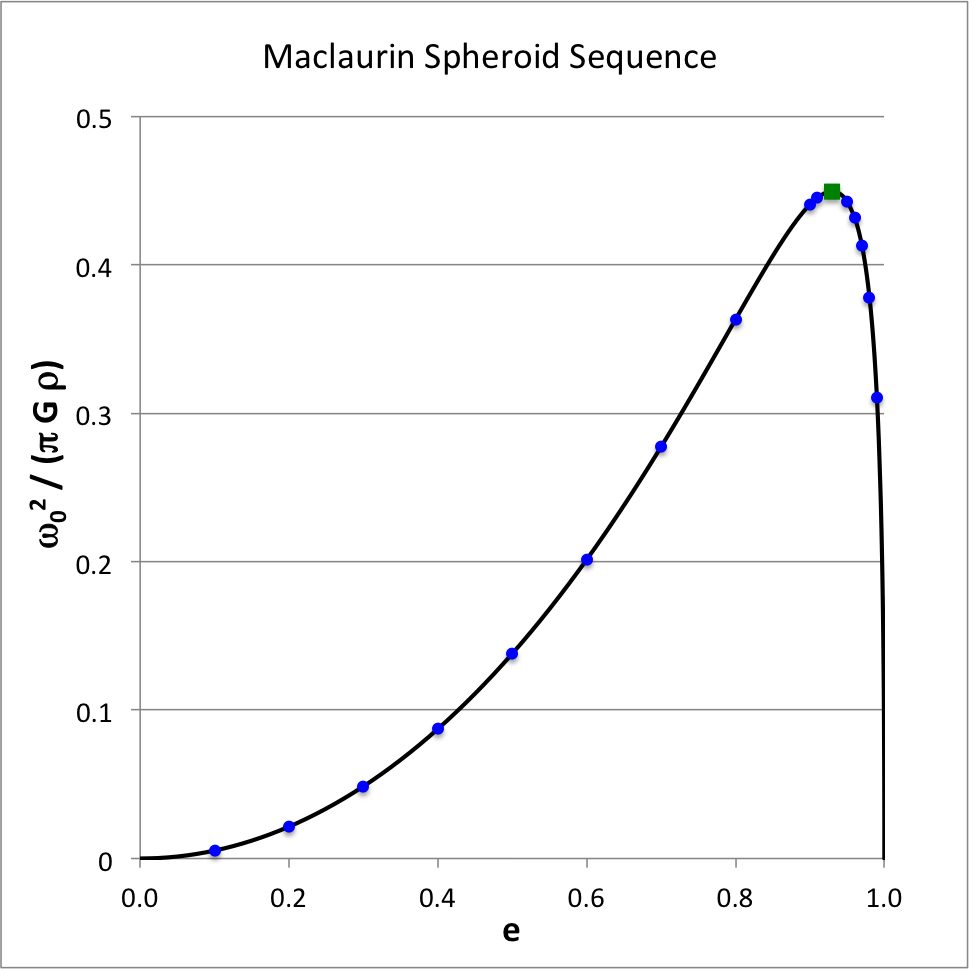The dark blue circular markers locate 15 of the 18 individual models identified in Table 1. The solid black curve derives from our evaluation of the function, $~\omega_0^2(e)$; this curve also may be found in: Fig. 5 (p. 79) of [EFE]; Fig. 7.2 (p. 173) of [ST83]

The essential structural elements of each Maclaurin spheroid model are uniquely determined once we specify the system's axis ratio, $~c/a$, or the system's meridional-plane eccentricity, $~e$, where

 $~e$ $~\equiv$ $~\biggl[1 - \biggl(\frac{c}{a}\biggr)^2\biggr]^{1 / 2} \, ,$

which varies from e = 0 (spherical structure) to e = 1 (infinitesimally thin disk). According to our accompanying derivation, for a given choice of $~e$, the square of the system's equilibrium angular velocity is,

 $~ \omega_0^2$ $~=$ $2\pi G \rho \biggl[ A_1 - A_3 (1-e^2) \biggr] \, ,$ [EFE], §32, p. 77, Eq. (4) [T78], §4.5, p. 86, Eq. (52) [ST83], §7.3, p. 172, Eq. (7.3.18)

where,

 $~A_1$ $~=$ $\frac{1}{e^2} \biggl[\frac{\sin^{-1}e}{e} - (1-e^2)^{1/2} \biggr](1-e^2)^{1/2} \, ,$ $~A_3$ $~=$ $\frac{2}{e^2} \biggl[(1-e^2)^{-1/2} -\frac{\sin^{-1}e}{e} \biggr](1-e^2)^{1/2} \, .$ Thomson & Tait (1867), §522, p. 392, Eqs. (9) & (7) [EFE], §17, p. 43, Eq. (36) [T78], §4.5, p. 85, Eqs. (48) & (49) [ST83], §7.3, p. 170, Eq. (7.3.8)
 Table 1Data copied fromThomson & Tait (1867), §772, p. 614 $~e$ $~\frac{\omega_0^2}{2\pi G \rho}$ $~e$ $~\frac{\omega_0^2}{2\pi G \rho}$ 0.10 0.0027 0.91 0.2225 0.20 0.0107 0.92 0.2241 0.30 0.0243 0.93 0.2247 0.40 0.0436 0.94 0.2239 0.50 0.0690 0.95 0.2213 0.60 0.1007 0.96 0.2160 0.70 0.1387 0.97 0.2063 0.80 0.1816 0.98 0.1890 0.90 0.2203 0.99 0.1551

In other words,

 $~ \frac{\omega_0^2}{2\pi G \rho }$ $~=$ $(3-2e^2)(1-e^2)^{1 / 2} \cdot \frac{\sin^{-1}e}{e^3} - \frac{3(1-e^2)}{e^2} \, .$ Thomson & Tait (1867), §771, p. 613, Eq. (1) H. Lamb (orig. 1879)], 2nd Ed. (1895), Ch. XII, §313, p. 583, Eq. (7) — set $~\zeta^2 = (1-e^2)/e^2$ G. H. Darwin (1886), p.322, Eq. (14) — set $~\gamma = \sin^{-1}e$ J. H. Jeans (1928), §192, p. 202, Eq. (192.4) [EFE], §32, p. 78, Eq. (6) [ST83], §7.3, p. 172, Eq. (7.3.18)

Figure 1 shows how the square of the angular velocity varies with eccentricity along the Maclaurin spheroid sequence; given the chosen normalization unit, $~\pi G\rho$, it is understood that the density of the configuration is held fixed as the eccentricity is varied.

 Examining the Maclaurin spheroid sequence "… we see that the value of $~\omega_0^2$ increases gradually from zero to a maximum as the eccentricity $~e$ rises from zero to about 0.93, and then (more quickly) falls to zero as the eccentricity rises from 0.93 to unity." … "If the angular velocity exceed the value associated with this maximum, "… equilibrium is impossible in the form of an ellipsoid of revolution. If the angular velocity fall short of this limit there are always two ellipsoids of revolution which satisfy the conditions of equilibrium. In one of these the eccentricity is greater than 0.93, in the other less." ---Thomson & Tait (1867), §772, p. 614.

In our Figure 1, the small solid-green square marker identifies the location along the sequence where the system with the maximum angular velocity resides:

 $~\biggl[ e, \frac{\omega_0^2}{\pi G \rho} \biggr]$ $~\equiv$ $~\biggl[ 0.92995, 0.449331 \biggr] \, .$ [EFE], §32, p. 80, Eqs. (9) & (10)

### Corresponding Total Angular Momentum

 Figure 2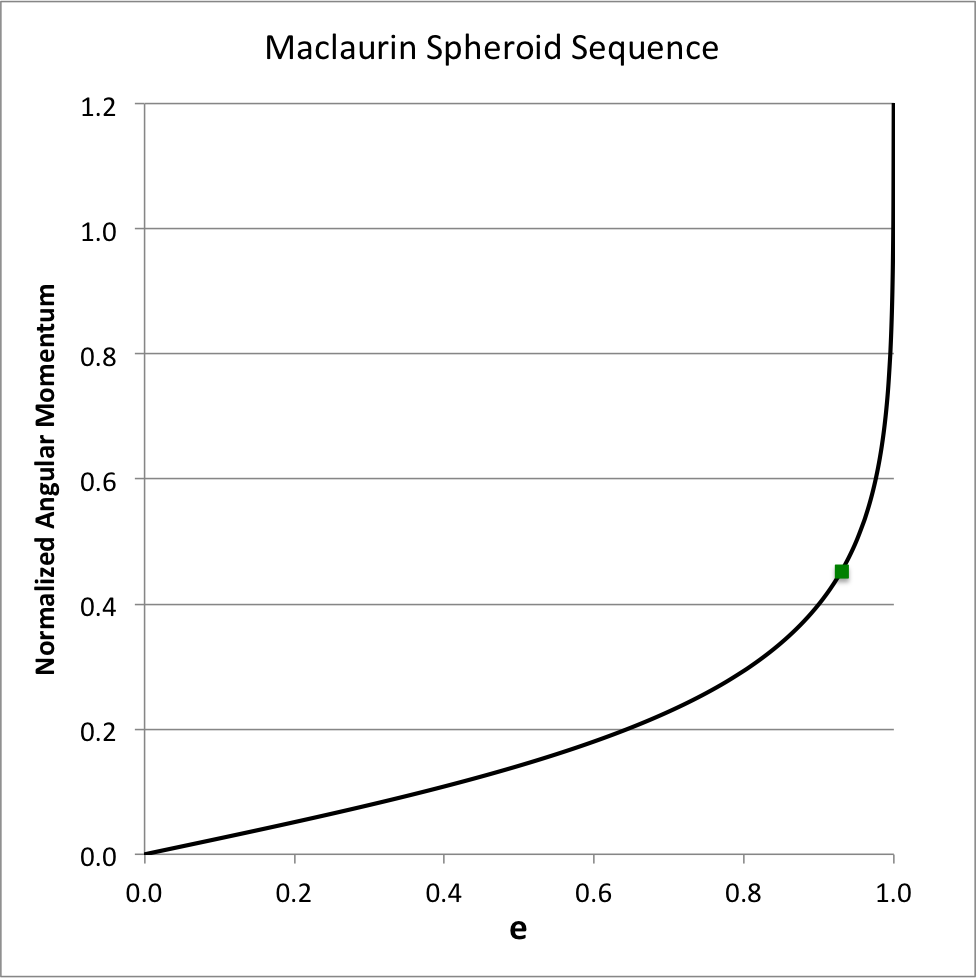Solid black curve also may be found as: Fig. 6 (p. 79) of [EFE]; Fig. 7.3 (p. 174) of [ST83]

The total angular momentum of each uniformly rotating Maclaurin spheroid is given by the expression,

 $~L$ $~=$ $~I \omega_0 \, ,$

where, the moment of inertia $~(I)$ and the total mass $~(M)$ of a uniform-density spheroid are, respectively,

 $~I$ $~=$ $~\biggl(\frac{2}{5}\biggr) M a^2 \, ,$ and, $~M$ $~=$ $~\biggl( \frac{4\pi}{3} \biggr) \rho a^2c \, .$

Hence, we have,

 $~L^2$ $~=$ $~ \frac{2^2 M^2 a^4}{5^2} \biggl[ A_1 - A_3 (1-e^2) \biggr] 2\pi G \biggl[ \frac{3}{2^2\pi} \cdot \frac{M}{a^2c} \biggr]$ $~=$ $~ \frac{6GM^3 {\bar{a}}}{5^2} \biggl[ A_1 - A_3 (1-e^2) \biggr]\biggl(\frac{a}{c}\biggr)^{4/3}$ $~\Rightarrow ~~~ \frac{L}{(GM^3\bar{a})^{1 / 2}}$ $~=$ $~ \frac{6^{1 / 2}}{5} \biggl[ A_1 - A_3 (1-e^2) \biggr]^{1 / 2}(1 - e^2)^{-1 / 3} \, ,$ [EFE], §32, p. 78, Eq. (7) [T78], §4.5, p. 86, Eq. (54) where,         $~\bar{a} \equiv (a^2 c)^{1 / 3} \, .$

Figure 2 shows how the system's angular momentum varies with eccentricity along the Maclaurin spheroid sequence; given the chosen normalization unit, $~(GM^3\bar{a})^{1 / 2}$, it is understood that the mass and the volume — hence, also the density — of the configuration are held fixed as the eccentricity is varied. Strictly speaking, along this sequence the angular momentum asymptotically approaches infinity as $~e \rightarrow 1$; by limiting the ordinate to a maximum value of 1.2, the plot masks this asymptotic behavior. The small solid-green square marker identifies the location along this sequence where the system with the maximum angular velocity resides (see Figure 1); this system is not associated with a turning point along this angular-momentum versus eccentricity sequence.

## Alternate Sequence Diagrams

### Energy Ratio, T/|W|

 Table 2:  Limiting Values $~e=0$ $~e=1$ $~A_1$ $\frac{2}{3}$ $~0$ $~A_3$ $\frac{2}{3}$ $~2$ $~\frac{\sin^{-1}e}{e}$ $~1$ $~\frac{\pi}{2}$ $~\tau \equiv \frac{T_\mathrm{rot}}{|W_\mathrm{grav}|}$ $~0$ $~\frac{1}{2}$

The rotational kinetic energy of each uniformly rotating Maclaurin spheroid is given by the expression,

 $~T_\mathrm{rot}$ $~=$ $~ \frac{1}{2}I \omega_0^2 =\frac{Ma^2}{5} \cdot 2\pi G\rho \biggl[ A_1 - (1-e^2)A_3\biggr]$ $~=$ $~ \frac{2^3 \pi^2}{3\cdot 5} \cdot G\rho^2 a^4 c \biggl[ A_1 - (1-e^2)A_3\biggr]$ $~=$ $~ \frac{2^3\pi^2}{3\cdot 5} \cdot G\rho^2 a^5 \biggl[ \frac{(1-e^2)}{e^3} ~(3 - 2e^2)\sin^{-1}e - \frac{3(1-e^2)^{3 / 2}}{e^2} \biggr] \, ;$

and the gravitational potential energy of each configuration is,

 $~W_\mathrm{grav}$ $~=$ $~ - \frac{3}{5} \cdot \frac{GM^2}{c} \biggl[ A_1 + \frac{1}{2}(1-e^2)A_3 \biggr] = - \frac{3}{2\cdot 5} \cdot \frac{G}{c} \biggl[ \frac{2^2\pi \rho a^2 c}{3} \biggr]^2 \biggl[ 2A_1 + (1-e^2)A_3 \biggr]$ $~=$ $~ - \frac{2^3\pi^2}{3\cdot 5}\cdot G\rho^2 a^4 c \biggl[ 2A_1 + (1-e^2)A_3 \biggr]$ $~=$ $~ - \frac{2^4 \pi^2}{3\cdot 5} \cdot G\rho^2 a^5 (1-e^2) \cdot \frac{\sin^{-1}e }{e} \, .$

Hence, the energy ratio,

 $~\tau \equiv \frac{T_\mathrm{rot}}{|W_\mathrm{grav}|}$ $~=$ $~ \frac{ A_1 - (1-e^2)A_3 }{ 2A_1 + (1-e^2)A_3 }$ [T78], §4.5, p. 86, Eq. (53) $~=$ $~ \biggl[ \frac{(1-e^2)}{e^3} ~(3 - 2e^2)\sin^{-1}e - \frac{3(1-e^2)^{3 / 2}}{e^2} \biggr] \biggl[ 2(1-e^2) \cdot \frac{\sin^{-1}e }{e} \biggr]^{-1}$ $~=$ $~ \frac{3}{2e^2}\biggl[ 1 - \frac{e(1-e^2)^{1 / 2}}{\sin^{-1} e}\biggr] - 1 \, .$ [ST83], §7.3, p. 172, Eq. (7.3.24) [P00], Vol. I, §10.3, p. 489, Eq. (10.54)

Building on an accompanying discussion of the structure of Maclaurin spheroids, Table 2 — shown just above, on the right — lists the limiting values of several key functions. Note, in particular, that as the eccentricity varies smoothly from zero (spherical configuration) to unity (infinitesimally thin disk), the energy ratio, $~\tau$, varies smoothly from zero to one-half. In his examination of the Maclaurin spheroid sequence, Tassoul (1978) chose to use this energy ratio as the order parameter, rather than the eccentricity.

 Figure 3 Figure 4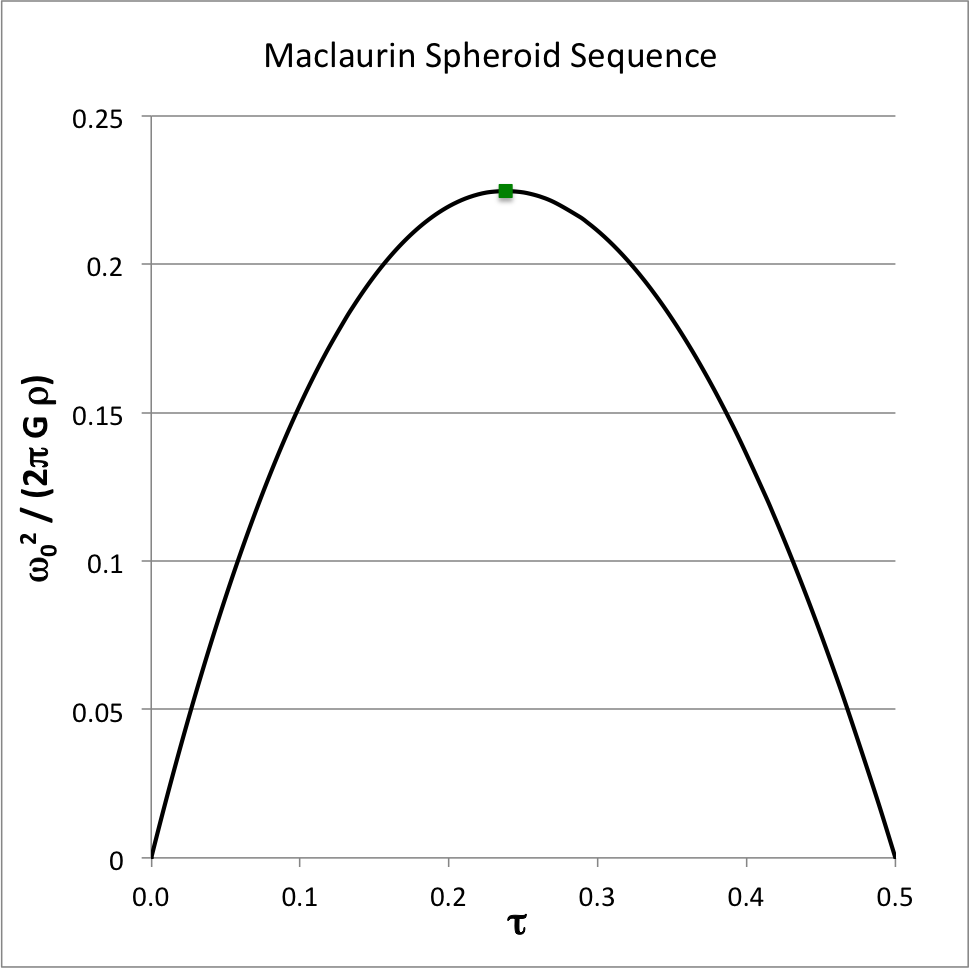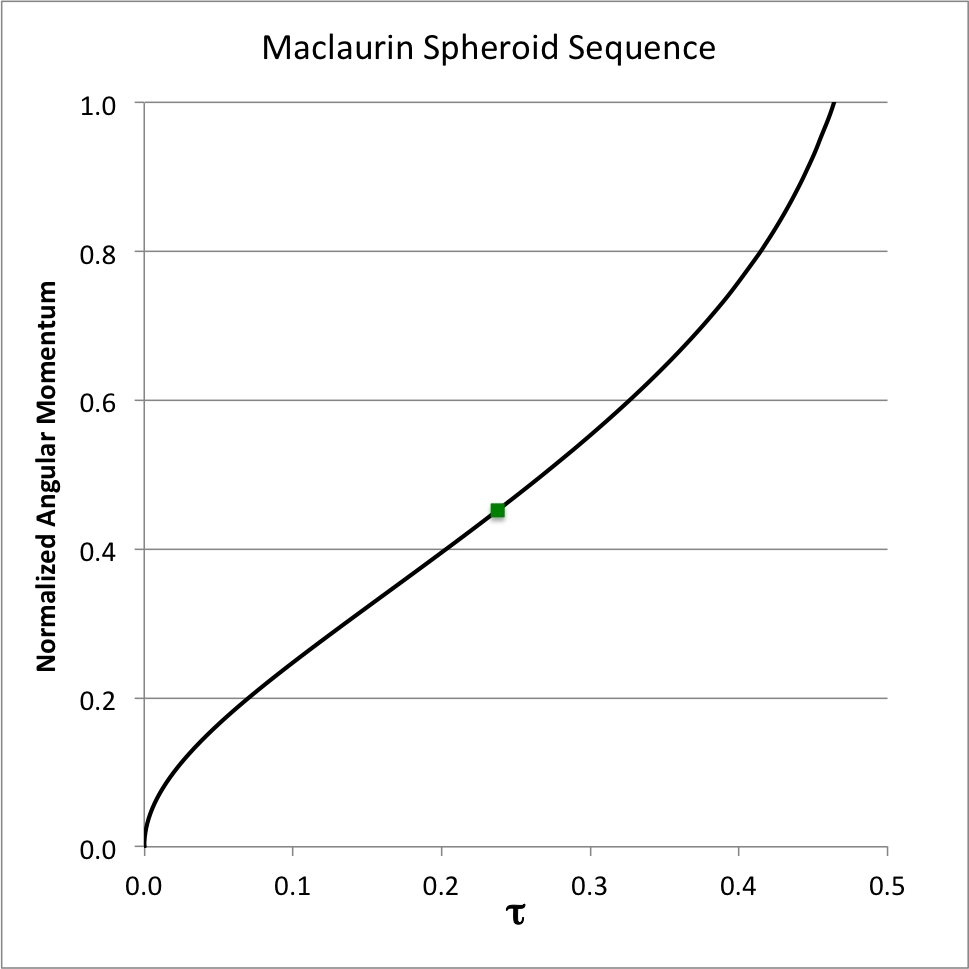Solid black curve also may be found in: Fig. 4.2 (p. 88) & Fig. 10.1 (p. 236) of [T78] This solid black curve also appears in: Fig. 4.2 (p. 88) & Fig. 10.12 (p. 237) of [T78]

Following Tassoul, our Figure 3 shows how the square of the angular velocity varies with $~\tau$, and our Figure 4 shows how the system angular momentum varies with $~\tau$. In these plots, respectively, the square of the angular velocity has been normalized by $~2\pi G \rho$ — that is, by a quantity that is a factor of two larger than the normalization adopted in EFE — while the angular momentum has been normalized to the same quantity used in EFE. As above, the small solid-green square marker identifies the location along the sequence where the system with the maximum angular velocity resides.

### Angular Velocity or T/|W| vs. Angular Momentum

Figures 5 and 6, respectively, show how the square of the angular velocity and how the energy ratio, τ, vary with the square of the angular momentum for models along the Maclaurin spheroid sequence. In generating these plots, following the lead of Eriguchi & Hachisu (1983), we have normalized the square of the angular velocity by $~4\pi G \rho$ — a factor of four larger than the normalization used in EFE — and we have adopted a slightly different angular-momentum normalization, namely,

 $~j$ $~\equiv$ $~ \biggl( \frac{3}{2^8 \pi^4} \biggr)^{1/6} \frac{L}{(GM^3\bar{a})^{1 / 2}} \, . $
 Figure 5 Figure 6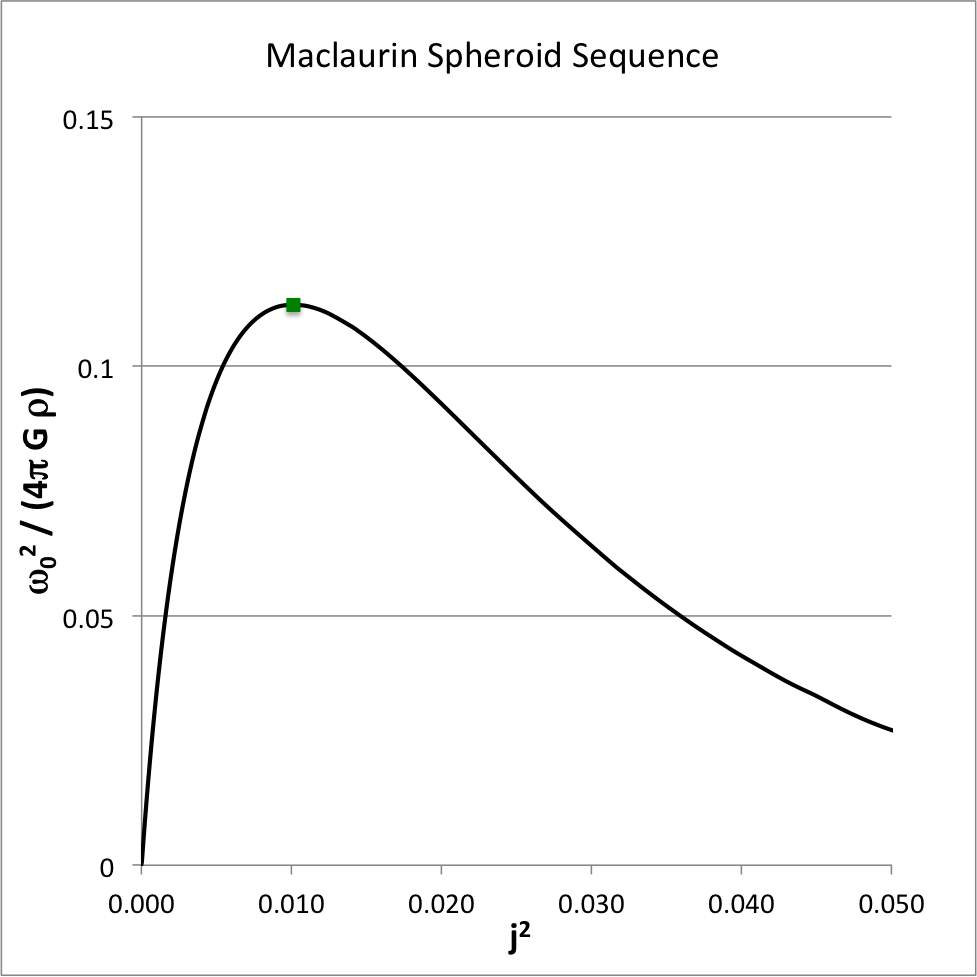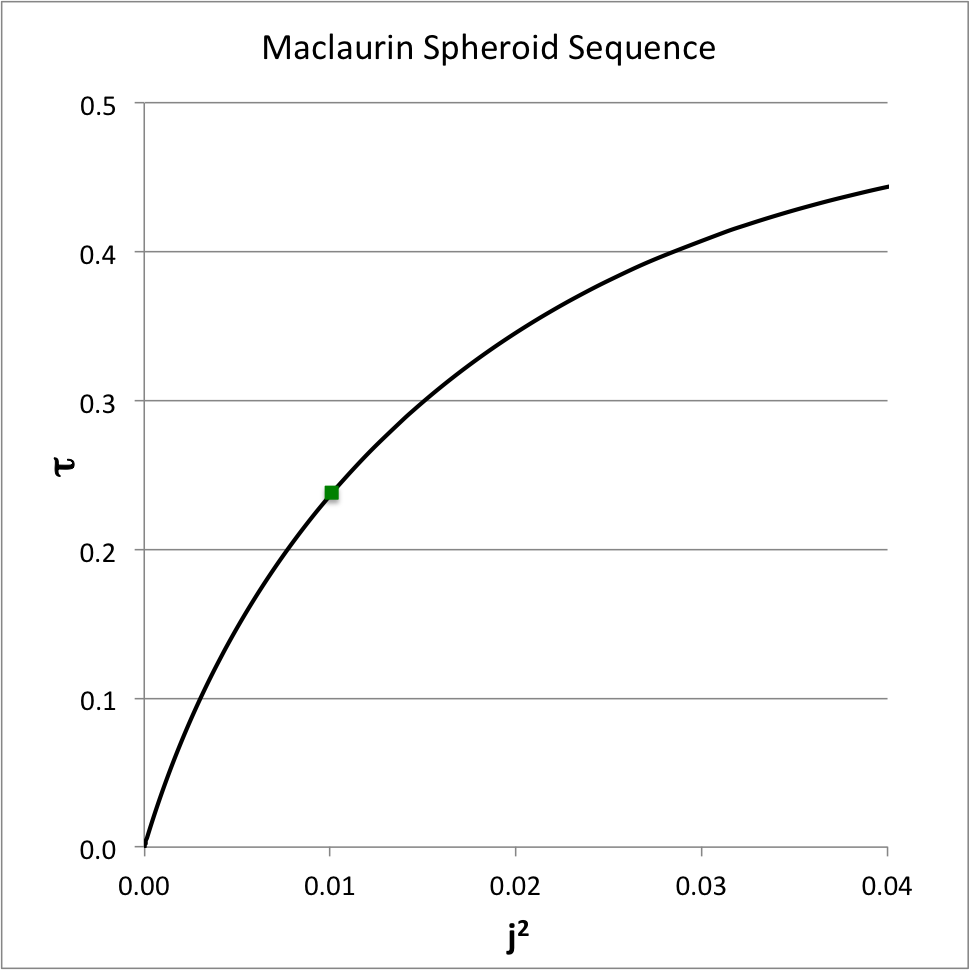This solid black curve also appears in: Fig. 3 (p. 1134) of Eriguchi & Hachisu (1983) Fig. 3 (p. 487) of Hachisu (1986) Fig. 4 (p. 4507) of Basillais & Huré (2019) This solid black curve also appears in: Fig. 4 (p. 487) of Hachisu (1986)

As above, the small solid-green square marker identifies the location along both sequences where the system with the maximum angular velocity resides:

 $~\biggl[ j^2, \frac{\omega_0^2}{4\pi G \rho}, \tau \biggr]$ $~\equiv$ $~\biggl[ 0.010105, 0.112333, 0.237894 \biggr] \, .$